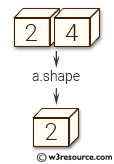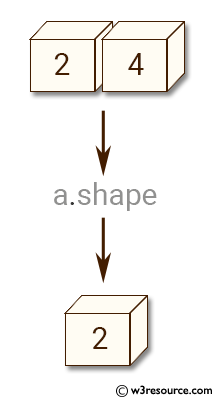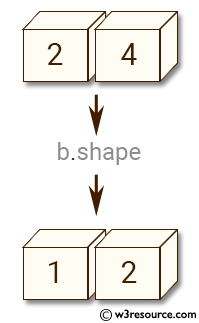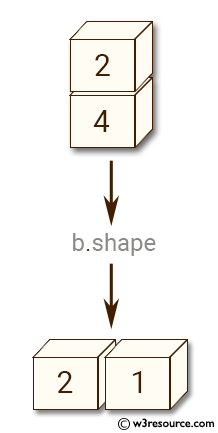# NumPy Array manipulation: expand_dims() function

## numpy.expand_dims() function

The expand_dims() function is used to expand the shape of an array.
Insert a new axis that will appear at the axis position in the expanded array shape.

Syntax:

`numpy.expand_dims(a, axis)`Version: 1.15.0

Parameter:

Name Description Required /
Optional
a Input array. Required
subok IPosition in the expanded axes where the new axis is placed. Required

Return value:

res [ndarray] Output array. The number of dimensions is one greater than that of the input array.

Example-1: numpy.expand_dims()

``````>>> import numpy as np
>>> a = np.array([2, 4])
>>> a.shape
(2,)
``````

Pictorial Presentation:Example-2: numpy.expand_dims()

``````>>> import numpy as np
>>> a = np.array([2, 4])
>>> b = np.expand_dims(a, axis=0)
>>> b
array([[2, 4]])
>>> b.shape
(1, 2)
``````

Pictorial Presentation:Example-3: numpy.expand_dims()

``````>>> import numpy as np
>>> b = np.expand_dims(a, axis=1) # Equivalent to x[:,np.newaxis]
>>> b
array([,
])
>>> b.shape
(2, 1)
``````

Pictorial Presentation:Example-4: numpy.expand_dims()

``````>>> import numpy as np
>>> np.newaxis is None
True
``````

Python - NumPy Code Editor: